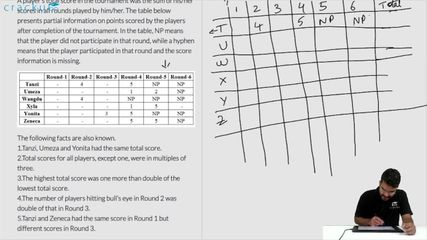### CAT 2019 Question Paper (Slot 1) Question 39

Instructions

Six players - Tanzi, Umeza, Wangdu, Xyla, Yonita and Zeneca competed in an archery tournament. The tournament had three compulsory rounds, Rounds 1 to 3. In each round every player shot an arrow at a target. Hitting the centre of the target (called bull’s eye) fetched the highest score of 5. The only other possible scores that a player could achieve were 4, 3, 2 and 1. Every bull’s eye score in the first three rounds gave a player one additional chance to shoot in the bonus rounds, Rounds 4 to 6. The possible scores in Rounds 4 to 6 were identical to the first three.

A player’s total score in the tournament was the sum of his/her scores in all rounds played by him/her. The table below presents partial information on points scored by the players after completion of the tournament. In the table, NP means that the player did not participate in that round, while a hyphen means that the player participated in that round and the score information is missing.

The following facts are also known.
1.Tanzi, Umeza and Yonita had the same total score.
2.Total scores for all players, except one, were in multiples of three.
3.The highest total score was one more than double of the lowest total score.
4.The number of players hitting bull’s eye in Round 2 was double of that in Round 3.
5.Tanzi and Zeneca had the same score in Round 1 but different scores in Round 3.

Question 39

# What was the highest total score?

Solution

It is given that every bull’s eye score in the first three rounds gave a player one additional chance to shoot in the bonus rounds, Rounds 4 to 6, which means Tanzi scored Bull's eye only once in the first 3 rounds because she participated only once in round 4 to 6. Similarly, Umeza scored Bull's eye exactly 2 times in the first 3 rounds. Wangdu did not score Bull's eye in the first three rounds and so on.

Now from 1, Tanzi, Umeza and Yonita had the same total score.

So, Total score of Tanzi will be 4+5+5+a=14+a,  (She scored Bull's eye(a score of 5) in exactly one round and a is the unknown score)

Total score of Umeza = 1+2+5+5+b = 13+b    (She scored Bull's eye(a score of 5) in exactly 2 rounds and b is the unknown score)

Total score of Yonita = 3+5+5+c=13+c (She scored Bull's eye(a score of 5) in exactly one round and c is the unknown score)

Now 14+a=13+b=13+c,

Also it is given that total scores for all players, except one, were in multiples of three, so these three will have to be a multiple of 3.

So, (a,b,c) can be either (1,2,2) or (4,5,5) in the same order. But the value (5,5) for b and c is not possible.  (Umeza scored Bull's eye in exactly 2 rounds and Yonita in exactly 1 round)

Hence, a=1,b=2 and c=2. So each of Tanzi, Umeza and Yonita had total score of 15.

Tabulating the data, we have

From 5, Tanzi and Zeneca had the same score in Round 1 but different scores in Round 3.

Zeneca score Bull's eye 2 times in round 1 to 3. If Tanzi scored 1 in round 1, then Zeneca also has to score 1 in round 1, which means both Tanzi and Zeneca scores in round 3 will be 5, which violates 5. Hence Tanzi scored 5 in round 1 and Zeneca also scored the same in round 1.So the new table is:

From 4, the number of players hitting bull’s eye in Round 2 was double of that in Round 3.

So, in round 3 either 1 or 2 Bull's eye can be scored and in round 2, 2 or 4 Bull's eye can be scored.

Case 1: If only 1 Bull's eye is scored in the round 3, then in round 3 Umeza will score 2 and Zeneca will score 2/3/4 in round 3, which means both will score 5 in round 2. So minimum Bull's eye in round 2 will be 3. (Umeza, Zeneca and Xyla)

Hence this case is rejected.

Case 2: 2 Bull's eye were scored in round 3 and 4 Bull's eye were scored in round 2. So in round 2 Umeza, Yonita and Zeneca scored 5. This can be tabulated as:

In round 3, 2 Bull's eye can only be scored by Xyla and Umeza.

The highest scorer can be either Xyla or Zeneca. The lowest scorer will be Wangdu.

1.Consider Zeneca is the highest scorer.

From 3, the highest total score was one more than double of the lowest total score. So the only possible score for Zeneca is 23 and that for Wangdu is 11. (11*2+1=23)

But this will violate condition 2, since both Zeneca and Wangdu do not have their scores as multiples of three in this case.

Hence, Xyla will be the highest scorer. The only possible total score for Xyla will be 25, and that for Wangdu is 12(4+4+4). (12*2+1=25)

Since Xyla already has non-multiple of 3 as total score. Zeneca will have 24 as the total score. The complete table is:

The highest score is 25.

### View Video Solution